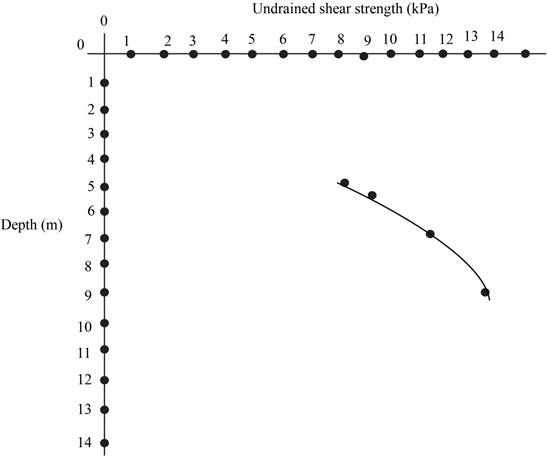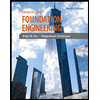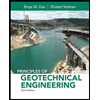#### Concept explainers

Textbook QuestionChapter 4, Problem 4.10QPP

A series of vane shear tests have been performed on a soft clay stratum. The results of these tests are as follows:The vane was 60 mm in diameter and 120 mm long. The soil has a liquid limit of 100 and a plastic limit of 30. Compute the undrained shear strength for each test, then develop a plot of undrained shear strength versus depth. This plot should have depth on the vertical axis, with zero at the top of the plot.07:44
Students have asked these similar questions
A field vane shear test was conducted in saturated clay. The height and diameter of the vane were 101.6 mm and 50.8 mm respectively. During the test, the maximum torque applied was 0.0168 kN.m Determine the undrained shear strength of the clay? The clay soil described above has a liquid limit of 64 and a plastic limit of 29. What is the plasticity index?
The following data are from a direct shear test on an undisturbed soil sample. Represent the data by a Mohr Circle and compute the minor principal stress. Normal pressure = 16.2 kN/m2; Tangential pressure = 14.4 kN/m2, Angle of internal friction = 24°; c = 7.2 kN/m2. Show diagram
Separate triaxial compression tests are performed on identical soil samples. The soil is saturated mixed soil. At failure, the respective minor and major principal stresses values are 10 kPa and 30 kPa for sample one, and 40 kPa and 70 KPa for sample two. From these data, determine the value for ∅ and the cohesion intercept C on the Mohr’s circle shear strength coordinate. Show all hand calculations and diagrams.

Find more solutions based on key concepts
Knowledge BoosterRecommended textbooks for you
•Principles of Foundation Engineering (MindTap Cou...
Civil Engineering
ISBN:9781337705028
Author:Braja M. Das, Nagaratnam Sivakugan
Publisher:Cengage LearningPrinciples of Geotechnical Engineering (MindTap C...
Civil Engineering
ISBN:9781305970939
Author:Braja M. Das, Khaled Sobhan
Publisher:Cengage Learning
•Principles of Foundation Engineering (MindTap Cou...
Civil Engineering
ISBN:9781337705028
Author:Braja M. Das, Nagaratnam Sivakugan
Publisher:Cengage LearningPrinciples of Geotechnical Engineering (MindTap C...
Civil Engineering
ISBN:9781305970939
Author:Braja M. Das, Khaled Sobhan
Publisher:Cengage Learning